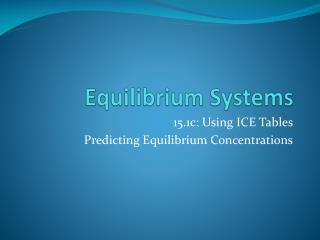Download PresentationEquilibrium Systems

# Equilibrium Systems - PowerPoint PPT PresentationDownload Presentation## Equilibrium Systems

- - - - - - - - - - - - - - - - - - - - - - - - - - - E N D - - - - - - - - - - - - - - - - - - - - - - - - - - -
##### Presentation Transcript

1. Equilibrium Systems 15.1c: Using ICE Tables Predicting Equilibrium Concentrations

2. Example 1 • At a certain temperature a 1.00 L flask initially contained 0.298 mol PCl3(g) and 8.70x10-3 mol PCl5(g). After the system had reached equilibrium, 2.00x10-3 mol Cl2(g) was found in the flask. PCl5(g)  PCl3(g) + Cl2(g) • Calculate the equilibrium concentrations of all the species and the value of K.

3. Example 1

4. Approximations • if K is very small, we can assume that the change (x) is going to be negligible • can be used to cancel out when adding or subtracting from a “normal” sized number • used to simplify algebra 0

5. Example 2 • At 35°C, K=1.6x10-5 for the reaction 2NOCl (g) ⇄ 2NO (g) + Cl2 (g) • Calculate the concentration of all species at equilibrium for the following mixtures • a) 2.0 mol NOCl in 2.0 L flask • b) 1.0 mol NOCl and 1.0 mol NO in 1.0 L flask • c) 2.0 mol NOCl and 1.0 mol Cl2 in 1.0 L flask

6. Example 2a • 2.0 mol NOCl in 2.0 L flask • [NOCl]=1.0 - (2 x 0.016)=0.97 M = 1.0 M, [NO]=0.032 M, [Cl2]=0.016 M

7. Example 2b • 1.0 mol NOCl and 1.0 mol NO in 1.0 L flask • [NOCl]=1.0 - (2x1.6x10-5)=0.999968 M = 1.0 M, [NO]=1.0 +(2x1.6x10-5)= 1.0 M, [Cl2]=1.6x10-5M

8. Example 2c • 2.0 mol NOCl and 1.0 mol Cl2 in 1.0 L flask • [NOCl]= 2.0 - (2x4.0x10-3)=1.992 M = 2.0 M, [Cl2]=1.0+4.0x10-3=1.0 M, [NO]=0.0080 M,

9. Homework Textbook: p 688 #6,9,10 LSM 15.1D